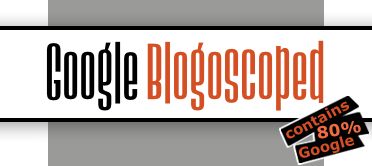### Sunday, June 27, 2004

### Mobile Communications and Collective Action by Howard Rheingold

“There are a number of gems in this presentation, which is overall very rousing about the possibilities of new technology and democratic empowerment. The one that made me think the most was the discussion of a UPC reader on a computer that looked the code up in a database and then did a Google search on that product, allowing you to know who things like whether the product was manufactured in an environmentally friendly way, if there was a store down the street that had it on sale, etc.”
– AKB, Howard Rheingold (Demand Media), April 19th, 2004

More at the Demandmedia.net video blog.

### Communicating with Computers by Alan Kay

You can view an inspiring talk by computer legend Alan Kay – inventor of the Dynabook, an early version of the laptop of today – on the history of software, computing, WYSIWYG, object-orientiation, and interface design. (Plus, Alan Kay is telling you why at Xerox PARC, bean bag chairs were used.)
It’s amazing to see Douglas Engelbart of the Augmentation Research Center at Stanford Research Institute (where close-by at Stanford University, Google was spawned later on) showing off mouse, collaborative desktop, windows, hypertext, hierarchical folders, and web cam – all in the 1960s. Also, you can find an introduction to Seymour Papert’s work; he’s the man who invented Logo and connected kids to computers.Google has become so important in everyday life, I was wondering what a Google school would look like. [Thanks to the free Prelinger film archives and their Duck and Cover.]

### Lean HTML

You can keep your HTML files small by putting layout details into a stylesheet. This approach can help search engines as well as your human visitors, but most of all it makes your web site highly maintainable.

View the introduction to writing HTML with CSS [WMV]*.

*I’m editing HTML and CSS using open-source freeware Netpadd.

Alex Stapleton figured out the code to calculate the checksum to grab the PageRank for any URL. [Thanks Jesse.]

``````<?php
/*
This code is released unto the public domain
*/

//unsigned shift right
function zeroFill(\$a, \$b)
{
\$z = hexdec(80000000);
if (\$z & \$a)
{
\$a = (\$a>>1);
\$a &= (~\$z);
\$a |= 0x40000000;
\$a = (\$a>>(\$b-1));
}
else
{
\$a = (\$a>>\$b);
}
return \$a;
}

function mix(\$a,\$b,\$c) {
\$a -= \$b; \$a -= \$c; \$a ^= (zeroFill(\$c,13));
\$b -= \$c; \$b -= \$a; \$b ^= (\$a<<8);
\$c -= \$a; \$c -= \$b; \$c ^= (zeroFill(\$b,13));
\$a -= \$b; \$a -= \$c; \$a ^= (zeroFill(\$c,12));
\$b -= \$c; \$b -= \$a; \$b ^= (\$a<<16);
\$c -= \$a; \$c -= \$b; \$c ^= (zeroFill(\$b,5));
\$a -= \$b; \$a -= \$c; \$a ^= (zeroFill(\$c,3));
\$b -= \$c; \$b -= \$a; \$b ^= (\$a<<10);
\$c -= \$a; \$c -= \$b; \$c ^= (zeroFill(\$b,15));

return array(\$a,\$b,\$c);
}

if(is_null(\$length)) {
\$length = sizeof(\$url);
}
\$a = \$b = 0x9E3779B9;
\$c = \$init;
\$k = 0;
\$len = \$length;
while(\$len >= 12) {
\$a += (\$url[\$k+0] +(\$url[\$k+1]<<8) +(\$url[\$k+2]<<16) +(\$url[\$k+3]<<24));
\$b += (\$url[\$k+4] +(\$url[\$k+5]<<8) +(\$url[\$k+6]<<16) +(\$url[\$k+7]<<24));
\$c += (\$url[\$k+8] +(\$url[\$k+9]<<8) +(\$url[\$k+10]<<16)+(\$url[\$k+11]<<24));
\$mix = mix(\$a,\$b,\$c);
\$a = \$mix; \$b = \$mix; \$c = \$mix;
\$k += 12;
\$len -= 12;
}

\$c += \$length;
switch(\$len)              /* all the case statements fall through */
{
case 11: \$c+=(\$url[\$k+10]<<24);
case 10: \$c+=(\$url[\$k+9]<<16);
case 9 : \$c+=(\$url[\$k+8]<<8);
/* the first byte of c is reserved for the length */
case 8 : \$b+=(\$url[\$k+7]<<24);
case 7 : \$b+=(\$url[\$k+6]<<16);
case 6 : \$b+=(\$url[\$k+5]<<8);
case 5 : \$b+=(\$url[\$k+4]);
case 4 : \$a+=(\$url[\$k+3]<<24);
case 3 : \$a+=(\$url[\$k+2]<<16);
case 2 : \$a+=(\$url[\$k+1]<<8);
case 1 : \$a+=(\$url[\$k+0]);
/* case 0: nothing left to add */
}
\$mix = mix(\$a,\$b,\$c);
/*-------------------------------------------- report the result */
return \$mix;
}

//converts a string into an array of integers containing the numeric value of the char
function strord(\$string) {
for(\$i=0;\$i<strlen(\$string);\$i++) {
\$result[\$i] = ord(\$string{\$i});
}
return \$result;
}
// http://www.example.com/ - Checksum: 6540747202
\$url = 'info:'.\$_GET['url'];
print("url:t{\$_GET['url']}n");
printf("ch:t6%un",\$ch);
?>``````

Once you calculated the checksum, you can dynamically find out the PageRank for a page by reading the values returned by the following URL: